EENS 2110 Mineralogy Tulane University Prof. Stephen A. Nelson Crystal Form, Zones, Crystal Habit

 Crystal Forms As stated at the end of the last lecture, the next step is to use the Miller Index notation to designate crystal forms.  A crystal form is a set of crystal faces that are related to each other by symmetry.  To designate a crystal form (which could imply many faces) we use the Miller Index, or Miller-Bravais Index notation enclosing the indices in curly braces, i.e. {101} or {11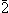1} Such notation is called a form symbol. An important point to note is that a form refers to a face or set of faces that have the same arrangement of atoms.  Thus, the number of faces in a form depends on the symmetry of the crystal. General Forms and Special Forms A general form is a form in a particular crystal class that contains faces that intersect all crystallographic axes at different lengths. It has the form symbol {hkl}  All other forms that may be present are called special forms.  In the monoclinic, triclinic, and orthorhombic crystal systems, the form {111} is a general form because in these systems faces of this form will intersect the a, b, and c axes at different lengths because the unit lengths are different on each axis.  In crystals of higher symmetry, where two or more of the axes have equal length, a general form must intersect the equal length axes at different multiples of the unit length.  Thus in the tetragonal system the form {121} is a general form.  In the isometric system a general form would have to be something like {123}. Open Forms and Closed Forms A closed form is a set of crystal faces that completely enclose space.  Thus, in crystal classes that contain closed forms, a crystal can be made up of a single form.An open form is one or more crystal faces that do not completely enclose space.  Example 1.  Pedions are single faced forms.  Since there is only one face in the form a pedion cannot completely enclose space.  Thus, a crystal that has only pedions, must have at least 3 different pedions to completely enclose space. Example 2.  A prism is a 3 or more faced form wherein the crystal faces are all parallel to the same line.  If the faces are all parallel then they cannot completely enclose space.  Thus crystals that have prisms must also have at least one additional form in order to completely enclose space. Example 3.  A dipyramid has at least 6 faces that meet in points at opposite ends of the crystal.  These faces can completely enclose space, so a dipyramid is closed form.  Although a crystal may be made up of a single dipyramid form, it may also have other forms present. In your textbook on pages 139 to 142, forms 1 through 18 are open forms, while forms 19 through 48 are closed forms. There are 48 possible forms that can be developed as the result of the 32 combinations of symmetry.  We here discuss some, but not all of these forms.
 Pedions A pedion is an open, one faced form.  Pedions are the only forms that occur in the Pedial class (1).  Since a pedion is not related to any other face by symmetry, each form symbol refers to a single face.  For example the form {100} refers only to the face (100), and is different from the form {00} which refers only to the face (00). Note that while forms in the Pedial class are pedions, pedions may occur in other crystal classes.
 Pinacoids A Pinacoid is an open 2-faced form made up of two parallel faces. In the crystal drawing shown here the form {111} is a pinacoid and consists of two faces, (111) and ().  The form {100} is also a pinacoid consisting of the two faces (100) and (00).  Similarly the form {010} is a pinacoid consisting of the two faces (010) and (00), and the form {001} is a two faced form consisting of the faces (001) and (00).  In this case, note that at least three of the above forms are necessary to completely enclose space.  While all forms in the Pinacoid class are pinacoids, pinacoids may occur in other crystal classes as well.Domes Domes are 2- faced open forms where the 2 faces are related to one another by a mirror plane.  In the crystal model shown here, the dark shaded faces belong to a dome.  The vertical faces along the side of the model are pinacoids (2 parallel faces).  The faces on the front and back of the model are not related to each other by symmetry, and are thus two different pedions.Sphenoids Sphenoids are2 - faced open forms where the faces are related to each other by a 2-fold rotation axis and are not parallel to each other.  The dark shaded triangular faces on the model shown here belong  to a sphenoid.  Pairs of similar vertical faces that cut the edges of the drawing are also pinacoids.  The top and bottom faces, however, are two different pedions.Prisms A prism is an open form consisting of three or more parallel faces.  Depending on the symmetry, several different kinds of prisms are possible.
 Trigonal prism:   3 - faced form with all faces parallel to a 3 -fold rotation axis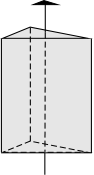Ditrigonal prism:  6 - faced form with all 6 faces parallel to a 3-fold rotation axis.  Note that the cross section of this form (shown to the right of the drawing) is not a hexagon, i.e. it does not have 6-fold rotational symmetry.Rhombic prism:  4 - faced form with all faces parallel to a line that is not a symmetry element.  In the drawing to the right, the 4 shaded faces belong to a rhombic prism.  The other faces in this model are pinacoids (the faces on the sides belong to a side pinacoid, and the faces on the top and bottom belong to a top/bottom pinacoid).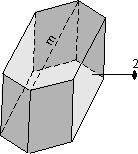Tetragonal prism: 4 - faced open form with all faces parallel to a 4-fold rotation axis or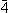.  The 4 side faces in this model make up the tetragonal prism.  The top and bottom faces make up the a form called the top/bottom pinacoid.Ditetragonal prism: 8 - faced form with all faces parallel to a 4-fold rotation axis.  In the drawing, the 8 vertical faces make up the ditetragonal prism.Hexagonal prism:  6 - faced form with all faces parallel to a 6-fold rotation axis. The 6 vertical faces in the drawing make up the hexagonal prism.  Again the faces on top and bottom are the top/bottom pinacoid form.Dihexagonal prism: 12 - faced form with all faces parallel to a 6-fold rotation axis. Note that a horizontal cross-section of this model would have apparent 12-fold rotation symmetry.  The dihexagonal prism is the result of mirror planes parallel to the 6-fold rotation axis.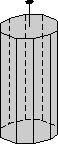Pyramids A pyramid is a 3, 4, 6, 8 or 12  faced open form where all faces in the form meet, or could meet if extended, at a point.
 Trigonal pyramid:  3-faced form where all faces are related by a 3-fold rotation axis.Ditrigonal pyramid: 6-faced form where all faces are related by a 3-fold rotation axis. Note that if viewed from above, the ditrigonal pyramid would not have a hexagonal shape; its cross section would look more like that of the trigonal prism discussed above.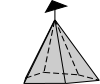Rhombic pyramid: 4-faced form where the faces are related by mirror planes. In the drawing shown here the faces labeled "p" are the four faces of the rhombic pyramid. If extend, these 4 faces would meet at a point.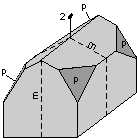Tetragonal pyramid: 4-faced form where the faces are related by a 4 axis. In the drawing the small triangular faces that cut the corners represent the tetragonal pyramid. Note that if extended, these 4 faces would meet at a point.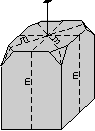Ditetragonal pyramid: 8-faced form where all faces are related by a 4 axis.  In the drawing shown here, the upper 8 faces belong to the ditetragonal pyramid form.  Note that the vertical faces belong to the ditetragonal prism.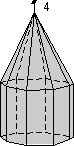Hexagonal pyramid: 6-faced form where all faces are related by a 6 axis. If viewed from above, the hexagonal pyramid would have a hexagonal shape.Dihexagonal pyramid: 12-faced form where all faces are related by a 6-fold axis. This form results from mirror planes that are parallel to the 6-fold axis.Dipyramids Dipyramids are closed forms consisting of 6, 8, 12, 16, or 24 faces.  Dipyramids are pyramids that are reflected across a mirror plane.  Thus, they occur in crystal classes that have a mirror plane perpendicular to a rotation or rotoinversion axis.
 Trigonal dipyramid: 6-faced form with faces related by a 3-fold axis with a perpendicular mirror plane. In this drawing, all six faces belong to the trigonal-dipyramid.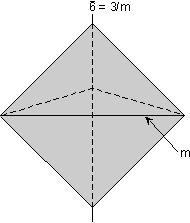Ditrigonal -dipyramid: 12-faced form with faces related by a 3-fold axis with a perpendicular mirror plane. If viewed from above, the crystal will not have a hexagonal shape, rather it would appear similar to the horizontal cross-section of the ditrigonal prism, discussed above.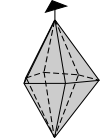Rhombic dipyramid:  8-faced form with faces related by a combinations of 2-fold axes and mirror planes.  The drawing to the right shows 2 rhombic dipyramids.  One has the form symbol {111} and consists of the four larger faces shown plus four equivalent faces on the back of the model.  The other one has the form symbol {113} and consists of the 4 smaller faces shown plus the four on the back.Tetragonal dipyramid: 8-faced form with faces related by a 4-fold axis with a perpendicular mirror plane.  The drawing shows the 8-faced tetragonal dipyramid.  Also shown is the 4-faced tetragonal prism, and the 2-faced top/bottom pinacoid.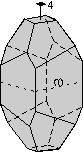Ditetragonal dipyramid: 16-faced form with faces related by a 4-fold axis with a perpendicular mirror plane. The ditetragonal dipyramid is shown here.  Note the vertical faces belong to a ditetragonal prism.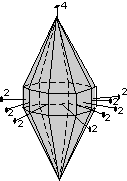Hexagonal dipyramid: 12-faced form with faces related by a 6-fold axis with a perpendicular mirror plane.  The vertical faces in this model make up a hexagonal prism.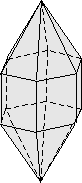Dihexagonal dipyramid: 24-faced form with faces related by a 6-fold axis with a perpendicular mirror plane.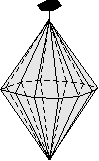Trapezohedrons Trapezohedron are closed 6, 8, or 12 faced forms, with 3, 4, or 6 upper faces offset from 3, 4, or 6 lower faces.  The trapezohedron results from 3-, 4-, or 6-fold axes combined with a perpendicular 2-fold axis.  An example of a tetragonal trapezohedron is shown in the drawing to the right. Other examples are shown in your textbook.Scalenohedrons A scalenohedron is a closed form with 8 or 12 faces.  In ideally developed faces each of the faces is a scalene triangle.  In the model, note the presence of the 3-fold rotoinversion axis perpendicular to the 3 2-fold axes.Rhombohedrons A rhombohedron is 6-faced closed form wherein 3 faces on top are offset by 3 identical upside down faces on the bottom, as a result of a 3-fold rotoinversion axis.  Rhombohedrons can also result from a 3-fold axis with perpendicular 2-fold axes.  Rhombohedrons only occur in the crystal classes2/m , 32, and.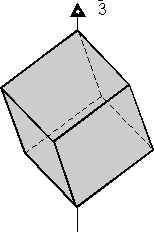Disphenoids A disphenoid is a closed form consisting of 4 faces.  These are only present in the orthorhombic system (class 222) and the tetragonal system (class)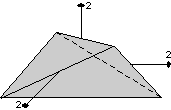The rest of the forms all occur in the isometric system, and thus have either four 3-fold axes or fouraxes.  Only some of the more common isometric forms will be discussed here.

 Hexahedron A hexahedron is the same as a cube.  4-fold axes are perpendicular to the face of the cube, and fouraxes run through the corners of the cube. Note that the form symbol for a hexahedron is {100}, and it consists of the following 6 faces:  (100), (010), (001), (00), (00), and (00).Octahedron An octahedron is an 8 faced form that results form three 4-fold axes with perpendicular mirror planes.  The octahedron has the form symbol {111}and consists of the following 8 faces: (111),  (), (11), (1), (1), (1), (11), and (11).  Note that four 3-fold axes are present that are perpendicular to the triangular faces of the octahedron (these 3-fold axes are not shown in the drawing).Dodecahedron A dodecahedron is a closed 12-faced form.  Dodecahedrons can be formed by cutting off the edges of a cube.  The form symbol for a dodecahedron is {110}.  As an exercise, you figure out the Miller Indices for these 12 faces.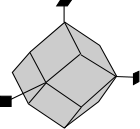Tetrahexahedron The tetrahexahedron is a 24-faced form with a general form symbol of {0hl} This means that all faces are parallel to one of the a axes, and intersect the other 2 axes at different lengths.Trapezohedron An isometric trapezohedron is a 12-faced closed form with the general form symbol {hhl}.  This means that all faces intersect two of the a axes at equal length and intersect the third a axis at a different length.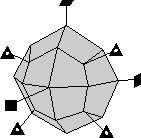Tetrahedron The tetrahedron occurs in the class3m and has the form symbol {111}(the form shown in the drawing) or {11} (2 different forms are possible).  It is a four faced form that results form threeaxes and four 3-fold axes (not shown in the drawing).Gyroid A gyroid is a form in the class 432 (note no mirror planes)Pyritohedron The pyritohedron is a 12-faced form that occurs in the crystal class 2/m.  Note that there are no 4-fold axes in this class.  The possible forms are {h0l} or {0kl} and each of the faces that make up the form have 5 sides.Diploid The diploid is the general form {hkl} for the diploidal class (2/m).  Again there are no 4-fold axes.Tetartoid Tetartoids are general forms in the tetartoidal class (23) which only has 3-fold axes and 2-fold axes with no mirror planes.Understanding Miller Indices, Form Symbols, and Forms In class we will fill in the following table in order to help you better understand the relationship between form and crystal faces.  The assignment will be to determine for each form listed across the top of the table the number of faces in that form, the name of the form, and the number of cleavage directions that the form symbol would imply for each of the crystal classes listed in the left-hand column.Before we can do this, however, we need to review how we define the crystallographic axes in relation to the elements of symmetry in each of the crystal systems.Triclinic - Since this class has such low symmetry there are no constraints on the axes, but the most pronounced face should be taken as parallel to the c axis.Monoclinic - The 2 fold axis is the b axis, or if only a mirror plane is present, the b axis is perpendicular to the mirror plane.
 Orthorhombic - The current convention is to take the longest axis as b, the intermediate axis is a, and the shortest axis is c.  An older convention was to take the c axis as the longest, the b axis intermediate, and the a axis as the shortest.Tetragonal - The c axis is either the 4 fold rotation axis or therotoinversion axis.Hexagonal - The c axis is the 6-fold, 3-fold,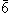axis, or.Isometric - The equal length a axes are either the 3  4-fold rotation axes,rotoinversion axes, or, in cases where no 4 oraxes are present, the 3 2-fold axes.

 Symmetry {010} {001} #Faces Form #Cleavage Directions #Faces Form #Cleavage Directions 1 2 2/m 2/m2/m2/m 4/m2/m2/m 4/m2/m

 Symmetry {110} {111} #Faces Form #Cleavage Directions #Faces Form #Cleavage Directions 1 2 2/m 2/m2/m2/m 4/m2/m2/m 4/m2/m

 Zones and Zone Symbols A zone is defined as a group of crystal faces that intersect in parallel edges.  Since the edges will all be parallel to a line, we can define that the direction of the line using a notation similar to Miller Indices.  This notation is called the zone symbol.  The zone symbol looks like a Miller Index, but is enclosed in square brackets, i.e. [uvw].  For a group of faces in the same zone, we can determine the zone symbol for all non-hexagonal minerals by choosing 2 non-parallel faces (hkl) and (pqr).
 To do so, we write the Miller Index for each face twice, one face directly beneath the other, as shown below.  The first and last numbers in each line are discarded.  Then we apply the following formula to determine the indices in the zone symbol. u  = k*r - l*q, v = l*p - h*r, and w = h*q - k*pFor example, faces (110) and (010) are not parallel to each other.  The zone symbol for these faces (and any other faces that lie in the same zone) is determined by writing 110 twice and then immediately below, writing 010 twice.  Applying the formula above gives the zone symbol for this zone as .Note that this zone symbol implies a line that is perpendicular to the face with the same index.  In other words,  is a line perpendicular to the face (001).  It can thus be used as a symbol for a line.  In this case, the line is the c crystallographic axis.
 Zone symbols, therefore are often used to denote directions through crystals. Being able to specify directions in crystals is important because many properties of minerals depend on direction.  These are called vectorial properties.Vectorial Properties of Crystals Although a crystal structure is an ordered arrangement of atoms on a lattice, as we have seen, the order may be different along different directions in the crystal.  Thus, some properties of crystals depend on direction.  These are called vectorial properties, and can be divided into two categories: continuous and discontinuous. Continuous Vectorial Properties Continuous vectorial properties depend on direction, but along any given the direction the property is the same.  Some of the continuous vectorial properties are: Hardness - In some minerals there is a difference in hardness in different directions in the crystal. Examples: Kyanite, Biotite, Muscovite.  This can become an important identifying property and/or may lead to confusion about the hardness if one is not aware of the directional dependence. Velocity of Light (Refractive Index) - For all minerals except those in the isometric system, the velocity of light is different as the light travels along different directions in the crystal.  We will use this directional dependence of light velocity as an important tool in the second half of the course.  Refractive Index is defined as the velocity of light in a vacum divided by the velocity of light in the material.  Because the velocity of light depends on direction, the refractive index will also depend on direction. Thermal Conductivity - The ability of a material to conduct heat is called thermal conductivity.  Like light, heat can be conducted at different rates along different directions in crystals. Electrical Conductivity-  The ability of a material to allow the passage of electrons is called electrical conductivity, which is also directionally dependent except in isometric crystals. Thermal Expansion - How much the crystal lattice expands as it is heated is referred to as thermal expansion.  Some crystals expand more in one direction than in others, thus thermal expansion is a vectorial property. Compressibility - Compressibility is a measure of how the lattice is reduced as atoms are pushed closer together under pressure.  Some directions in crystals may be more compressible than others. Discontinuous Vectorial Properties Discontinuous vectorial properties pertain only to certain directions or planes within a crystal.  For these kinds of properties, intermediate directions may have no value of the property.  Among the discontinuous vectorial properties are: Cleavage -  Cleavage is defined as a plane within the lattice along which breakage occurs more easily than along other directions.   A cleavage direction develops along zones of weakness in the crystal lattice.  Cleavage is discontinuous because it only occurs along certain planes. Growth Rate - Growth rate is defined as the rate at which atoms can be added to the crystal. In some directions fewer atoms must be added to the crystal than in other directions, and thus some directions may allow for faster growth than others.  Solution Rate - Solution rate is the rate at which a solid can be dissolved in a solvent.  In this case it depends on how tightly bonded the atoms are in the crystal structure, and this usually depends on direction.
 Crystal Habit In nature perfect crystals are rare.  The faces that develop on a crystal depend on the space available for the crystals to grow.  If crystals grow into one another or in a restricted environment, it is possible that no well-formed crystal faces will be developed.  However, crystals sometimes develop certain forms more commonly than others, although the symmetry may not be readily apparent from these common forms.  The term used to describe general shape of a crystal is habit. Some common crystal habits are as follows. Cubic - cube shapes Octahedral - shaped like octahedrons, as described above. Tabular -  rectangular shapes. Equant - a term used to describe minerals that have all of their boundaries of approximately equal length. Fibrous -  elongated clusters of fibers. Acicular -  long, slender crystals. Prismatic -  abundance of prism faces. Bladed -  like a wedge or knife blade Dendritic - tree-like growths Botryoidal - smooth bulbous shapes
 Examples of questions on this material that could be asked on an exam Define the following as they relate to crystal forms:  (a) Pedion, (b) pinacoid, (c) prism, (d) pyramid, (e) dipyramid, octahedrn, (f) dodechahedron. What is the difference between a closed form and an open form. What is a zone and what notation do we use to indicate a zone or a direction in a crystal? Given the symmetry of a crystal and a form symbol, be able to determine the number of faces that occur in the form (as in the example tables, above). What is the difference between a continuous vectorial property and a discontinuous vectorial property?  Give some examples of each. Return to EENS 2110 Page